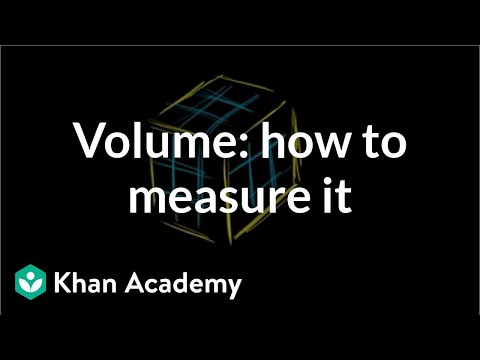# Measuring volume as area times length (Full video)

Description: Sal transitions from measuring volume with unit cubes to multiplying area times length. Created by Sal Khan. So I want to measure this volume in terms of cubic centimeters. We've already seen that we can do that by saying, hey, how many of these cubic centimeters can fit into this figure without them overlapping in any way? So I want to explore if we can somehow use these numbers to figure out how many of these cubic centimeters would fit into this figure.

### Other videos you might be interested in### Measuring volume with unit cubes (Full video)